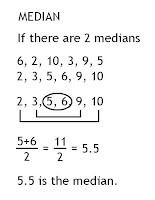### Arielle's Measures of Central Tendency

Monday, September 29, 2008

Mean: The mean is the sum of all data divided by the number of data points ; average.Median: The median is the middle value in a set of data. If there are 2 medians in the middle, add them together and divide by 2.Mode: The mode is the most frequently occurring number in a set data, there can be more than one mode.Range: The range is the difference between the largest and smallest values in a set of data.Here is a video that explains mean, median, mode, and range :